# Precalculus : Use Logarithms To Solve Exponential Equations and Inequalities

## Example Questions

### Example Question #1 : Exponential Equations And Inequalities

Solving an exponential equation.

Solve for,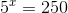.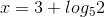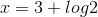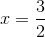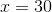Explanation:

We recall the property: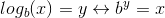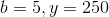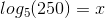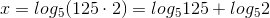Now,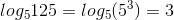.

Thus.

### Example Question #2 : Exponential Equations And Inequalities

Solving an exponential equation.

Solve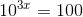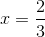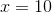Explanation:

Use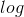(which is just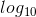, by convention) to solve.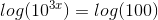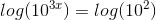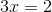.

### Example Question #3 : Exponential Equations And Inequalities

Solve the equation forusing the rules of logarithms.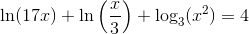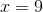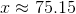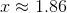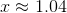Explanation:

Expanding the logarithms into sums of logarithms will cancel out the first two x terms, resulting in the equation: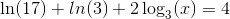Combining the first and second terms, then subtracting the new term over will allow you to isolate the variable term.

Divide both sides of the equation by 2, then exponentiate with 3.

Evaluating this term numerically will give the correct answer.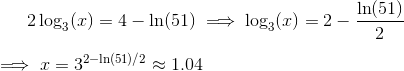### Example Question #4 : Exponential Equations And Inequalities

Solve the following equation: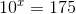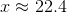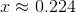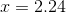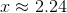Explanation:

To solve this equation, recall the following property: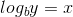Can be rewritten as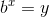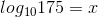Evaluate with your calculator to get### Example Question #5 : Exponential Equations And Inequalities

Solve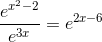.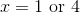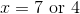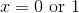Explanation:

After using the division rule to simplify the left hand side you can take the natural log of both sides.

If you then combine like terms you get a quadratic equation which factors to,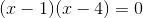.

Setting each binomial equal to zero and solving forwe get the solution to be.

### All Precalculus Resources